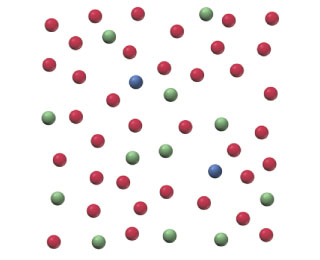# Problem: Below is a representation of 50 atoms of a fictitious element called westmontium (Wt). The red spheres represent Wt-296, the blue spheres Wt-297, and the green spheres Wt-298. Calculate the percent natural abundance of Wt-297 isotope.

###### FREE Expert Solution

We're asked to calculate the percent natural abundance of Wt-297 isotope.

We’re given a diagram showing 50 atoms of a fictitious element called westmontium (Wt):

• red spheres: Wt-296
• blue spheres: Wt-297
• green spheres: Wt-298

Recall that percent abundance is the percent value of the number of naturally occurring isotopes for a given element.  We will use the equation:

To do so, we need to count the # of Wt-297 isotopes from the given diagram:

95% (262 ratings)###### Problem Details

Below is a representation of 50 atoms of a fictitious element called westmontium (Wt). The red spheres represent Wt-296, the blue spheres Wt-297, and the green spheres Wt-298.Calculate the percent natural abundance of Wt-297 isotope.